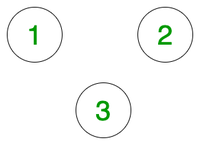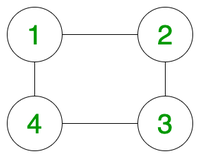# Find if there is a path between two vertices in an undirected graph

Given an undirected graph with N vertices and E edges and two vertices (U, V) from the graph, the task is to detect if a path exists between these two vertices. Print “Yes” if a path exists and “No” otherwise.

Examples:U = 1, V = 2
Output: No
Explanation:
There is no edge between the two points and hence its not possible to reach 2 from 1.

Input:U = 1, V = 3
Output: Yes
Explanation: Vertex 3 from vertex 1 via vertices 2 or 4.

## Recommended: Please try your approach on {IDE} first, before moving on to the solution.

Naive Approach:
The idea is to use Floyd Warshall Algorithm. To solve the problem, we cneed to try out all intermediate vertices ranging [1, N] and check:

1. If there is a direct edge already which exists between the two nodes.
2. Or we have a path from node i to intermediate node k and from node k to node j.

Below is the implementation of the above approach:

## C++

 `// C++ program to detect if a path ` `// exists between any two vertices ` `// for the given undirected graph ` `#include ` `using` `namespace` `std; ` ` `  `// Class representing a undirected ` `// graph using matrix ` `// representation ` `class` `Graph { ` `    ``int` `V; ` `    ``int``** g; ` ` `  `public``: ` `    ``Graph(``int` `V); ` ` `  `    ``// Function to add an edge to graph ` `    ``void` `addEdge(``int` `v, ``int` `w); ` ` `  `    ``// Function to check if ` `    ``// there exists a path or not ` `    ``bool` `isReachable(``int` `s, ``int` `d); ` ` `  `    ``// function to compute paths ` `    ``// in the matrix using ` `    ``// Floyd Warshall Algorithm ` `    ``void` `computePaths(); ` `}; ` ` `  `Graph::Graph(``int` `V) ` `{ ` `    ``this``->V = V; ` `    ``g = ``new` `int``*[V + 1]; ` `    ``for` `(``int` `i = 1; i < V + 1; i++) { ` `        ``// Rows may not be contiguous ` `        ``g[i] = ``new` `int``[V + 1]; ` ` `  `        ``// Initialize all entries ` `        ``// as false to indicate ` `        ``// that there are ` `        ``// no edges initially ` `        ``memset``(g[i], 0, (V + 1) * ``sizeof``(``int``)); ` `    ``} ` ` `  `    ``// Initializing node to itself ` `    ``// as it is always reachable ` `    ``for` `(``int` `i = 1; i <= V; i++) ` `        ``g[i][i] = 1; ` `} ` ` `  `// Function to add edge between nodes ` `void` `Graph::addEdge(``int` `v, ``int` `w) ` `{ ` `    ``g[v][w] = 1; ` `    ``g[w][v] = 1; ` `} ` ` `  `// Function to compute the path ` `void` `Graph::computePaths() ` `{ ` `    ``// Use Floyd Warshall algorithm ` `    ``// to detect if a path exists ` `    ``for` `(``int` `k = 1; k <= V; k++) { ` ` `  `        ``// Try every vertex as an ` `        ``// intermediate vertex ` `        ``// to check if a path exists ` `        ``for` `(``int` `i = 1; i <= V; i++) { ` `            ``for` `(``int` `j = 1; j <= V; j++) ` `                ``g[i][j] = g[i][j] ` `                          ``| (g[i][k] ` `                             ``&& g[k][j]); ` `        ``} ` `    ``} ` `} ` ` `  `// Function to check if nodes are reachable ` `bool` `Graph::isReachable(``int` `s, ``int` `d) ` `{ ` ` `  `    ``if` `(g[s][d] == 1) ` `        ``return` `true``; ` `    ``else` `        ``return` `false``; ` `} ` ` `  `// Driver code ` `int` `main() ` `{ ` ` `  `    ``Graph _g(4); ` `    ``_g.addEdge(1, 2); ` `    ``_g.addEdge(2, 3); ` `    ``_g.addEdge(1, 4); ` `    ``_g.computePaths(); ` ` `  `    ``int` `u = 4, v = 3; ` `    ``if` `(_g.isReachable(u, v)) ` `        ``cout << ``"Yes\n"``; ` `    ``else` `        ``cout << ``"No\n"``; ` ` `  `    ``return` `0; ` `} `

Output:

```Yes
```

Time Complexity: O(V3)
Auxiliary Space : O(V2)

Efficient Solution
We can either use BFS or DFS to find if there is a path from u to v. Below is a BFS based solution

## C++

 `// C++ program to check if there is exist a path between ` `// two vertices of an undirected graph. ` `#include ` `#include ` `using` `namespace` `std; ` ` `  `// This class represents an undirected graph ` `// using adjacency list representation ` `class` `Graph { ` `    ``int` `V; ``// No. of vertices ` ` `  `    ``// Pointer to an array containing adjacency lists ` `    ``list<``int``>* adj;  ` `public``: ` `    ``Graph(``int` `V); ``// Constructor ` ` `  `    ``// function to add an edge to graph ` `    ``void` `addEdge(``int` `v, ``int` `w);  ` `    ``bool` `isReachable(``int` `s, ``int` `d); ` `}; ` ` `  `Graph::Graph(``int` `V) ` `{ ` `    ``this``->V = V; ` `    ``adj = ``new` `list<``int``>[V]; ` `} ` ` `  `void` `Graph::addEdge(``int` `v, ``int` `w) ` `{ ` `    ``adj[v].push_back(w); ` `    ``adj[w].push_back(v); ` `} ` ` `  `// A BFS based function to check whether d is reachable from s. ` `bool` `Graph::isReachable(``int` `s, ``int` `d) ` `{ ` `    ``// Base case ` `    ``if` `(s == d) ` `        ``return` `true``; ` ` `  `    ``// Mark all the vertices as not visited ` `    ``bool``* visited = ``new` `bool``[V]; ` `    ``for` `(``int` `i = 0; i < V; i++) ` `        ``visited[i] = ``false``; ` ` `  `    ``// Create a queue for BFS ` `    ``list<``int``> queue; ` ` `  `    ``// Mark the current node as visited and enqueue it ` `    ``visited[s] = ``true``; ` `    ``queue.push_back(s); ` ` `  `    ``// it will be used to get all adjacent vertices of a vertex ` `    ``list<``int``>::iterator i; ` ` `  `    ``while` `(!queue.empty()) { ` `        ``// Dequeue a vertex from queue and print it ` `        ``s = queue.front(); ` `        ``queue.pop_front(); ` ` `  `        ``// Get all adjacent vertices of the dequeued vertex s ` `        ``// If a adjacent has not been visited, then mark it ` `        ``// visited  and enqueue it ` `        ``for` `(i = adj[s].begin(); i != adj[s].end(); ++i) { ` ` `  `            ``// If this adjacent node is the destination node,  ` `            ``// then return true ` `            ``if` `(*i == d) ` `                ``return` `true``; ` ` `  `            ``// Else, continue to do BFS ` `            ``if` `(!visited[*i]) { ` `                ``visited[*i] = ``true``; ` `                ``queue.push_back(*i); ` `            ``} ` `        ``} ` `    ``} ` ` `  `    ``// If BFS is complete without visiting d ` `    ``return` `false``; ` `} ` ` `  `// Driver program to test methods of graph class ` `int` `main() ` `{ ` `    ``// Create a graph given in the above diagram ` `    ``Graph g(4); ` `    ``g.addEdge(0, 1); ` `    ``g.addEdge(0, 2); ` `    ``g.addEdge(1, 2); ` `    ``g.addEdge(2, 0); ` `    ``g.addEdge(2, 3); ` `    ``g.addEdge(3, 3); ` ` `  `    ``int` `u = 1, v = 3; ` `    ``if` `(g.isReachable(u, v)) ` `        ``cout << ``"\n There is a path from "` `<< u << ``" to "` `<< v; ` `    ``else` `        ``cout << ``"\n There is no path from "` `<< u << ``" to "` `<< v; ` ` `  `    ``return` `0; ` `} `

Output:

```There is a path from 1 to 3
```

Time Complexity: O(V + E)
Auxiliary Space: O(V)

Attention reader! Don’t stop learning now. Get hold of all the important DSA concepts with the DSA Self Paced Course at a student-friendly price and become industry ready.

My Personal Notes arrow_drop_upCheck out this Author's contributed articles.

If you like GeeksforGeeks and would like to contribute, you can also write an article using contribute.geeksforgeeks.org or mail your article to contribute@geeksforgeeks.org. See your article appearing on the GeeksforGeeks main page and help other Geeks.

Please Improve this article if you find anything incorrect by clicking on the "Improve Article" button below.